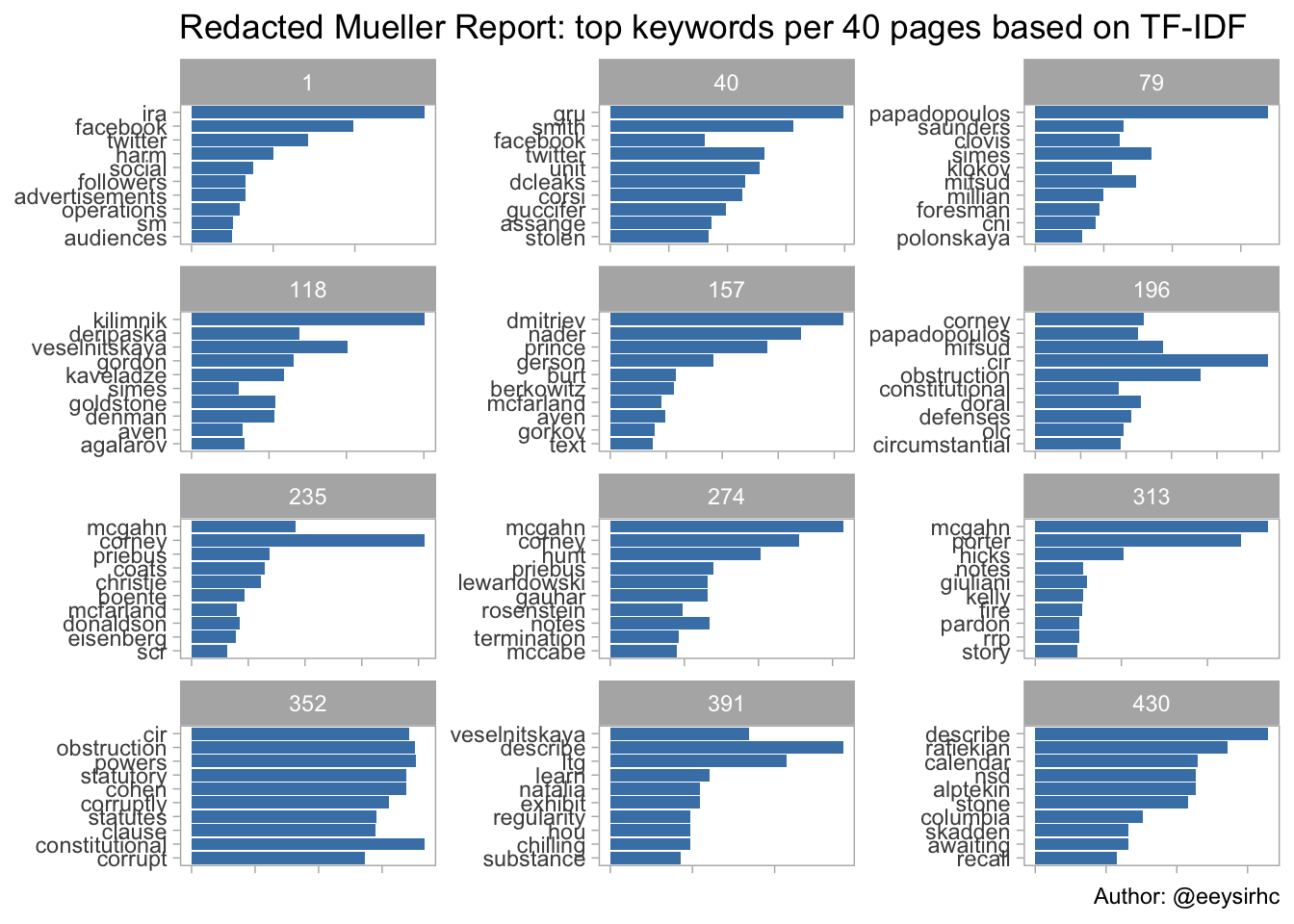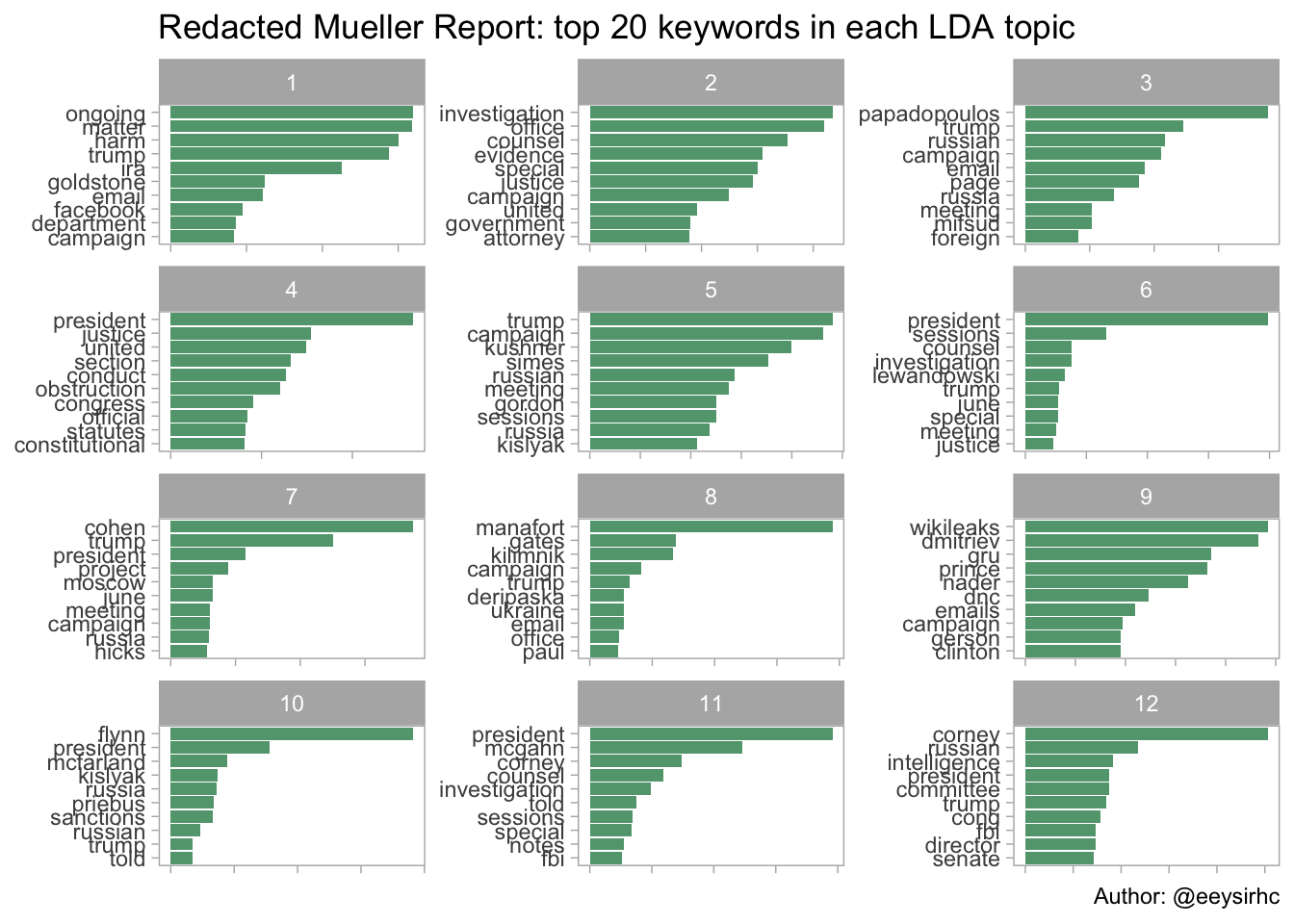After two politically-charged years, Robert Mueller finally concluded his investigation on Russian interference with the 2016 presidential elections.

The outcome was a 440+ page report on their findings - the perfect candidate for some text mining.

Side note: the idea for this post came when my attempts to extract the PDF text proved unsuccessful because it was locked in an unsearchable version.

As a consequence of that I did a little tweet mining instead:

Forutnately there are people much smarter than me and were able to produce a user-friendly format of the redacted report.

## Get the data

``````library(tidyverse)
library(tidytext)

mueller_report <- mueller_report_raw``````

## Clean the data

``````# REMOVE NON-CHAR AND NON-NUMBERS
mueller_report\$text <- str_replace_all(mueller_report\$text,"[^a-zA-Z\\s]", " ")

# CONVERT TO LOWER CASE
mueller_report\$text <- str_to_lower(mueller_report\$text)

# REMOVE EXTRA WHITE SPACE
mueller_report\$text <- str_replace_all(mueller_report\$text,"[\\s]+", " ")``````

## Calculate and plot TF-IDF for every 40 pages

``````data(stop_words)

mueller_report %>%
mutate(page_group = 39 * (page %/% 39)+ 1,   # CREATE GROUPS PER 40 PAGES
page_group = as.factor(page_group)) %>%
group_by(page_group) %>%
unnest_tokens(word, text) %>%
anti_join(stop_words) %>%
count(word, sort = TRUE) %>%
bind_tf_idf(word, page_group, n) %>%
arrange(desc(tf_idf)) %>%
group_by(page_group) %>%
top_n(10) %>%
ungroup() %>%
mutate(word = reorder(word, tf_idf)) %>%
ggplot() +
geom_col(aes(word, tf_idf),
show.legend = FALSE,
fill = 'steelblue') +
facet_wrap(~page_group, ncol = 3, scales = "free") +
coord_flip() +
theme_light() +
theme(panel.grid.major = element_blank(),
panel.grid.minor = element_blank(),
axis.text.x = element_blank()) +
labs(x = NULL,
y = NULL,
title = "Redacted Mueller Report: top keywords per 40 pages based on TF-IDF",
caption = "Author: @eeysirhc")``````Following each page group gives an idea of the history, events and conclusions from the Mueller report.

## Create the document term matrix for topic modeling

``````library(topicmodels)

desc_dtm <- mueller_report %>%
group_by(page) %>%
unnest_tokens(word, text) %>%
anti_join(stop_words) %>%
count(word, sort = TRUE) %>%
cast_dtm(page, word, n)

desc_lda <- LDA(desc_dtm, k = 12, control = list(seed = 1234))   # 12 WAS BEST FIT AFTER I TRIED 9 AND 24

top_terms <- tidy(desc_lda) %>%
group_by(topic) %>%
top_n(10, beta) %>%
ungroup() %>%
arrange(topic, -beta)``````

## Process and plot the LDA topic models

``````top_terms %>%
mutate(term = reorder(term, beta)) %>%
group_by(topic, term) %>%
arrange(desc(beta)) %>%
ungroup() %>%
mutate(term = factor(paste(term, topic, sep = "__"),
levels = rev(paste(term, topic, sep = "__")))) %>%
ggplot(aes(term, beta)) +
geom_col(show.legend = FALSE, fill = 'seagreen', alpha = 0.8) +
coord_flip() +
scale_x_discrete(labels = function(x) gsub("__.+\$", "", x)) +
labs(title = "Redacted Mueller Report: top 20 keywords in each LDA topic",
x = NULL, y = NULL,
caption = "Author: @eeysirhc") +
facet_wrap(~ topic, ncol = 3, scales = "free") +
theme_light() +
theme(panel.grid.major = element_blank(),
panel.grid.minor = element_blank(),
axis.text.x = element_blank())``````With latent Dirichlet allocation, or LDA, we now have a sense of the major topics and the associated keywords in the redacted report.

In other words (no pun intended), we are now speed reading with data science.